Divisor Drips andSquare Root Waves Prime Numbers are the Holes in Complex Composite Number Patterns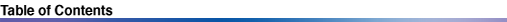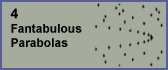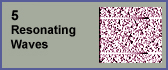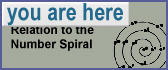6. Relation to the Number Spiral The Ulam Spiral is a graph of the prime numbers arranged in a rectangular spiral. Diagonal patterns can be seen throughout the spiral .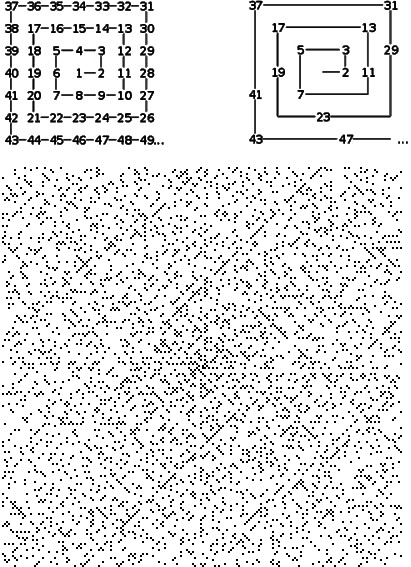Robert Sacks discovered another way to arrange the primes: in a circular spiral. Below at left is an illustration showing the construction of the number spiral . I lifted it from Sacks' web site ( www.numberspiral.com ). The number spiral is described as follows: roll the number line like a ribbon counterclockwise such that the perfect squares (0, 1, 4, 9, 16...) line up at right. This is shown in the illustration below at the upper-left. To the right of that is shown the first 2026 numbers as dots. The bottom of the illustration shows the patterns among the first 2026 primes, and the first 46656 primes in the number spiral.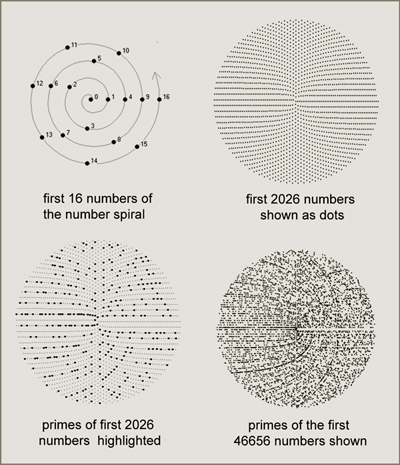I decided to try the inverse of showing the primes, and instead to show composite numbers, plotted here with gray values determined by the divisor function d(x) for all numbers x in the spiral. In this illustration, white indicates d(x) = 2 (primes), and black indicates d(x) = 20 or more. This is another way to visualize the idea that primes are just holes in overlapping composite number patterns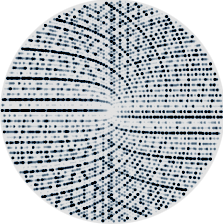Product Curves In his web site, Sacks shows several remarkable properties of number spirals. For instance, he describes a series of continuous functions that can be mapped as curves onto the spiral. The first curve, shown in the illustration below labeled "S" contains the perfect squares.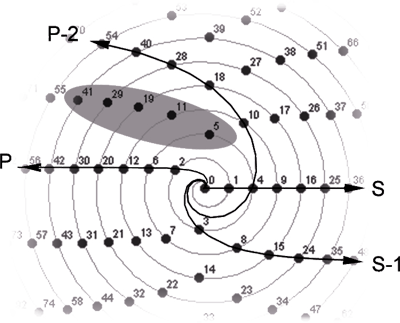It is the only straight curve. The second curve, labeled, "P", shoots off to the left, in the opposite direction as the S curve. It traverses the pronics (products of divisors with a difference of 1). The curve which contains numbers that are the products of divisors with a difference of 2 is shown just below the S curve. It is called the "S-1 curve". The curve which contains numbers which are the products of divisors with a difference of 3 is called the "P-2" curve. Notice the cluster of primes (5, 11, 19, 29, and 41) lying on a curve in-between the P and P-2 curves. This is just one of the many prime-rich curves that extend outward (and tending leftward) in the number spiral. Now let's take a look at how these curves relate to the divisor plot. The S curve corresponds to the square root boundary and it traverses the vertices of the square root parabolas. In the illustration below, the S curve has one of its perfect squares (5 = �25) highlighted with a circle. Notice that the divisor plot has pairs of curves corresponding to all of the curves in the number spiral. The S curve is the only one that does not map to a double-curve in the divisor plot. Four examples of x (24, 25, 28, and 30) have their divisor pairs indicated with circles - this is meant to emphasize the fact that all of Sacks' product curves refer to divisor pairs. And here is something interesting about the cluster of primes we just saw on the number spiral: each one of those prime numbers is followed by a pronic number, and in the illustration below there is a corresponding gap immediately to the left of the pronics in the divisor plot.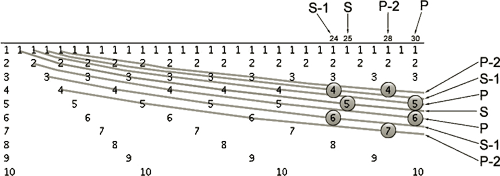We could continue mapping the product curves from the number spiral onto the divisor plot as shown - forever. It appears that every divisor pair would be visited by a pair of product curves. Now it's time for a new way to classify divisors: let's refer to a pair of product curves on the divisor plot as Cn, where n of 0 corresponds to the square root boundary, and where increasingly higher values of n correspond to pairs of product curves that are increasingly farther away from the square root boundary. An algorithm for specifying Cn (provided by Sacks) is as follows: y1 = (n+2 x i)/2 - n/2 y2 = (n+2 x i)/2 + n/2 x = y1 x y2; ....where n is the difference between divisors, and, for each n, i is an integer that varies from 0 to infinity. Another way to describe these curves is with the following equation (also provided by Sacks)...Notice in the image above that the divisors lying on each product curve increase by 1 as the curve extends to the right. They comprise an ordinal set of integers. We have seen a similar property with two other structures: zero reflection rays, and square root parabolas. Now we have a third way to organize divisors as sets of ordinal integers in the divisor plot. We will look at all of these ordinal curves in the next section. Rolling the Divisor Plot onto the Number Spiral The relationships between the number spiral and the divisor plot are numerous. And in fact, Rob Sacks had done preliminary explorations similar to mine before working on the number spiral. Just as the number spiral takes the 1-dimensional number line and coils it up to occupy a disk, the divisor plot (a 2D graph) likewise can be rolled up like a scroll to occupy a cylindrical volume, corresponding to the number spiral, as illustrated below. Notice in the illustration below that the divisor plot is inverted so that the dense area is in contact with the number spiral.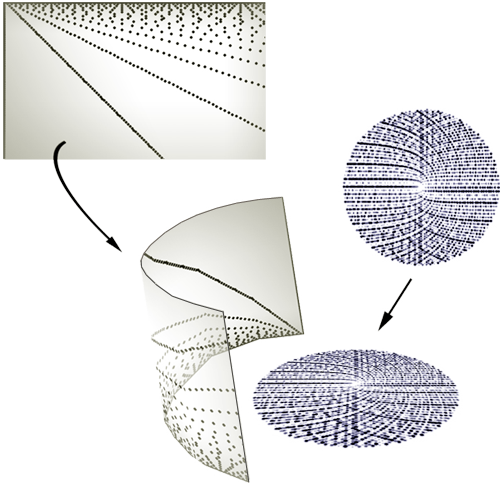Now let's look at the rolled-up divisor plot in 3D. The image below shows this volume rolled into a number spiral with 20000 numbers. Consider this as a 3D extension of the image above of the divisor function on the 2D number spiral. Vertical density of divisors in the 3rd dimension maps to color density in the 2D image.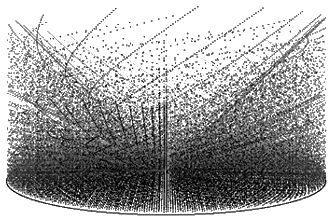To help reduce clutter, I plotted only divisors less than the square root of x. However, it is still rather dense. So I decided to plot only conjugate pairs of divisors whose difference is less than or equal to 16. This is shown below. This illustration reveals the fact that the square root boundary, when rolled onto the number spiral, conforms to a cone. It also makes the S-curve and P-curve more clearly visible (they are lying at the left-most and right-most edges of the cone).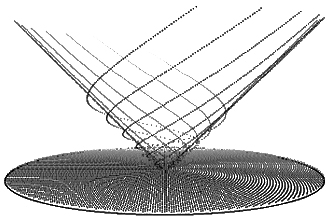Notice the similarity to one of Sacks' illustration of some of the product curves: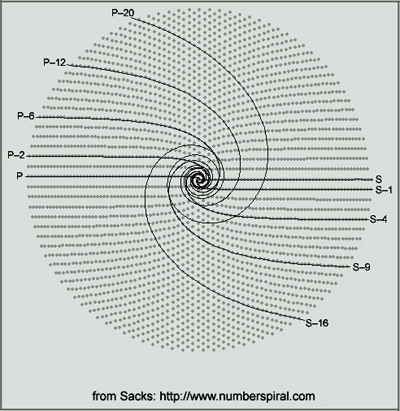The next image shows only the divisors for the S-curve less than or equal to the square root of x. Notice the pattern in the triangle that looks like a piece of the divisor plot. But in fact, this pattern does not occur in the divisor plot. In the divisor plot, these Dx would be spread out and intermittent among many other Dx. The regularities in this pattern show some similarities among square numbers - interesting, eh? Something else to explore some day.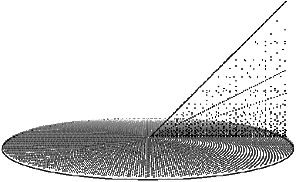The next image shows the end of the square root parabola P200, whose vertex is at (10000, 100). It appears distorted because it is conforming to a curved surface.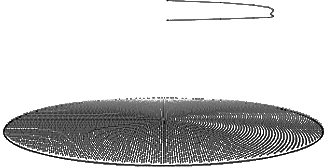The number spiral is a canvas for visualizing regularities that occur along the number line, which are not easily noticed when viewed along a straight line. Like all things circular and spiral, the number spiral reveals periodic features - which the divisor plot is full of. There are likely more discoveries to be made by plotting divisors on the number spiral in new ways.